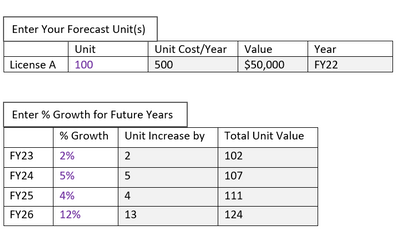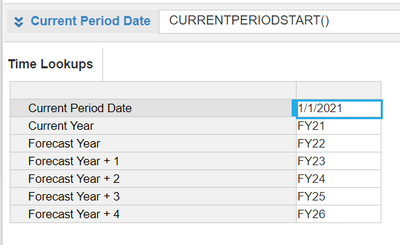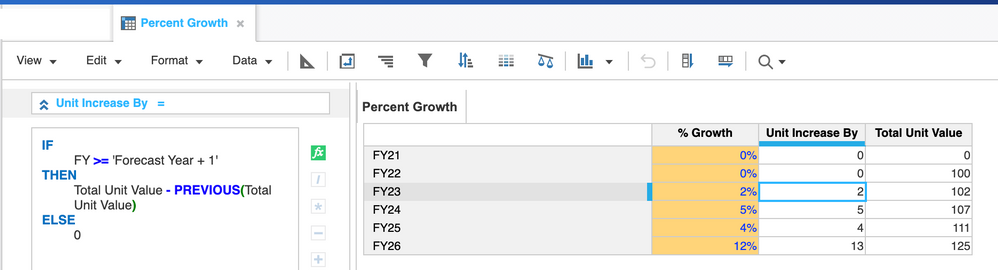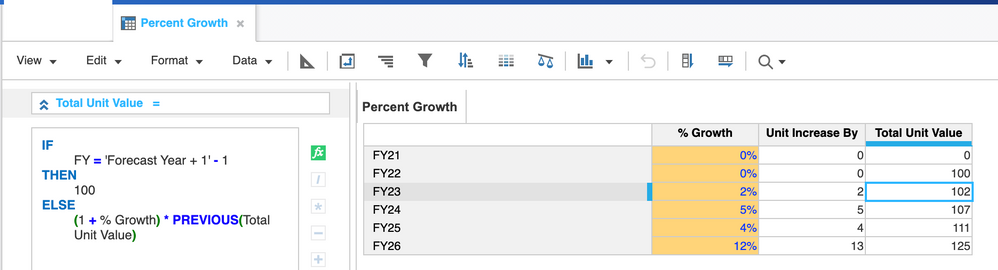# Percent Growth Formula LogicHello, everyone,

I'm trying to put together the formula to display the appropriate Unit Increase by & Total Unit Value outputs in the table above. The logic should go thus:

The 2% growth for FY23 should use the 100 unit value from FY22 as its base. The formula would go thus: (2% of 100)

The 5% growth for FY24 should use the Total Unit Value outcome from the FY23 calculation as its base. The formula would go thus: (5% of 102)

The 4% growth for FY25 should use the Total Unit Value outcome from the FY24 calculation as its base. The formula would go thus: (4% of 107).

.... and so on

FYI, FY23 - FY26 is a time range in the Model. Also, they are included as line items in a Time Lookups ModuleI would apprepriate your perspective on how I should approach this.

Thank you.

Tagged:

•Hi

If your calculation module for Unit Increase by & Total Unit Value is dimensioned against model time year, you can leverage on PREVIOUS function to achieve your goal.

I did a sample below for your reference. I have checking condition here since your FY22 is an input as 100. You may not need to follow exactly what I tried to replicate, just to give you an idea how PREVIOUS function works. You can also refer to the link here for a detailed explanation on this function:

https://help.anaplan.com/36463075-85be-4bab-900b-cde5ba112a0f-PREVIOUSHope this helps.

Tingting